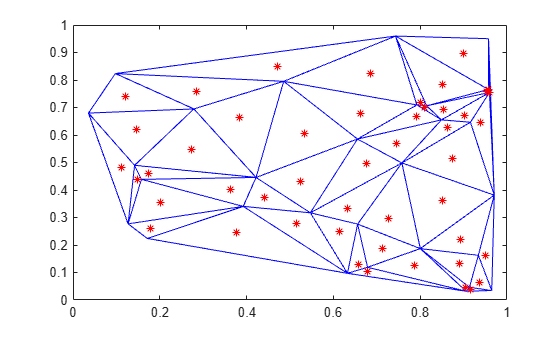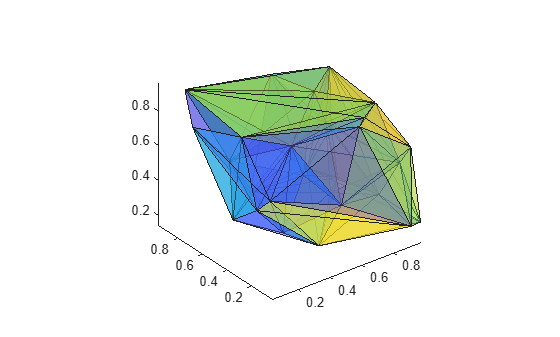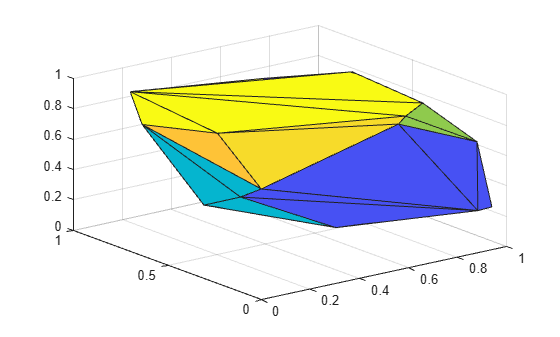# delaunayTriangulation

Delaunay triangulation in 2-D and 3-D

## Description

Use the `delaunayTriangulation` object to create a 2-D or 3-D Delaunay triangulation from a set of points. For 2-D data, you can also specify edge constraints.

You can perform a variety of topological and geometric queries on a `delaunayTriangulation`, including any `triangulation` query. For example, locate a facet that contains a specific point, find the vertices of the convex hull, or compute the Voronoi Diagram.

## Creation

To create a `delaunayTriangulation` object, use the `delaunayTriangulation` function with input arguments that define the triangulation's points and constrained edges.

### Syntax

``DT = delaunayTriangulation(P)``
``DT = delaunayTriangulation(P,C)``
``DT = delaunayTriangulation(x,y)``
``DT = delaunayTriangulation(x,y,C)``
``DT = delaunayTriangulation(x,y,z)``
``DT = delaunayTriangulation()``

### Description

example

````DT = delaunayTriangulation(P)` creates a Delaunay triangulation from the points in `P`. The matrix `P` has 2 or 3 columns, depending on whether your points are in 2-D or 3-D space.```
````DT = delaunayTriangulation(P,C)` specifies the edge constraints in the matrix `C` for the 2-D points in `P`. Each row of `C` defines the start and end vertex IDs of a constrained edge. Vertex IDs are the row numbers of the corresponding vertices in the property `DT.Points`.```
````DT = delaunayTriangulation(x,y)` creates a 2-D Delaunay triangulation from the point coordinates in the column vectors `x` and `y`.```
````DT = delaunayTriangulation(x,y,C)` specifies the edge constraints in a matrix `C`.```

example

````DT = delaunayTriangulation(x,y,z)` creates a 3-D Delaunay triangulation from the point coordinates in the column vectors `x`, `y`, and `z`. ```
````DT = delaunayTriangulation()` creates an empty Delaunay triangulation.```

### Input Arguments

expand all

Points, specified as a matrix whose columns are the x-coordinates, y-coordinates, and (possibly) `z`-coordinates of the triangulation points. The row numbers of `P` are the vertex IDs in the triangulation.

x-coordinates of triangulation points, specified as a column vector.

y-coordinates of triangulation points, specified as a column vector.

z-coordinates of triangulation points, specified as a column vector.

Vertex IDs of constrained edges, specified as a 2-column matrix. Each row of `C` corresponds to a constrained edge and contains two IDs:

• `C(j,1)` is the ID of the vertex at the start of an edge.

• `C(j,2)` is the ID of the vertex at end of the edge.

You can specify edge constraints for 2-D triangulations only.

## Properties

expand all

Points in the triangulation, represented as a matrix with the following characteristics:

• Each row in `DT.Points` contains the coordinates of a vertex.

• Each row number of `DT.Points` is a vertex ID.

Triangulation connectivity list, represented as a matrix with the following characteristics:

• Each element in `DT.ConnectivityList` is a vertex ID.

• Each row represents a triangle or tetrahedron in the triangulation.

• Each row number of `DT.ConnectivityList` is a triangle or tetrahedron ID.

Constrained edges, represented as a 2-column matrix of vertex IDs. Each row of `DT.Constraints` corresponds to a constrained edge and contains two IDs:

• `DT.Constraints(j,1)` is the ID of the vertex at the start of an edge.

• `DT.Constraints(j,2)` is the ID of the vertex at end of the edge.

`DT.Constraints` is an empty matrix when the triangulation has no constrained edges.

## Object Functions

 `convexHull` Convex hull of Delaunay triangulation `isInterior ` Query points inside Delaunay triangulation `voronoiDiagram` Voronoi diagram of Delaunay triangulation `barycentricToCartesian` Convert coordinates from barycentric to Cartesian `cartesianToBarycentric` Convert coordinates from Cartesian to barycentric `circumcenter` Circumcenter of triangle or tetrahedron `edgeAttachments` Triangles or tetrahedra attached to specified edge `edges` Triangulation edges `faceNormal` Triangulation unit normal vectors `featureEdges` Sharp edges of surface triangulation `freeBoundary` Free boundary facets `incenter` Incenter of triangulation elements `isConnected` Test if two vertices are connected by an edge `nearestNeighbor` Vertex closest to specified point `neighbors` Triangle or tetrahedron neighbors `pointLocation` Triangle or tetrahedron enclosing point `size` Size of triangulation connectivity list `vertexAttachments` Triangles or tetrahedra attached to vertex `vertexNormal` Triangulation vertex normal

## Examples

collapse all

Create a 2-D `delaunayTriangulation` object for 30 random points.

```rng default; P = rand([30 2]); DT = delaunayTriangulation(P)```
```DT = delaunayTriangulation with properties: Points: [30x2 double] ConnectivityList: [48x3 double] Constraints: [] ```

Compute the center points of each triangle, and plot the triangulation with the center points.

```IC = incenter(DT); triplot(DT) hold on plot(IC(:,1),IC(:,2),'*r')```Create a 3-D `delaunayTriangulation` object for 30 random points.

```rng default; x = rand([30 1]); y = rand([30 1]); z = rand([30 1]); DT = delaunayTriangulation(x,y,z)```
```DT = delaunayTriangulation with properties: Points: [30x3 double] ConnectivityList: [102x4 double] Constraints: [] ```

Plot the triangulation.

`tetramesh(DT,'FaceAlpha',0.3);`Compute and plot the convex hull of the triangulation.

```[K,v] = convexHull(DT); trisurf(K,DT.Points(:,1),DT.Points(:,2),DT.Points(:,3))```expand all

## Tips

• `delaunayTriangulation` can produce incorrect or inconsistent results when boundary constraints intersect or overlap. To avoid this behavior, use constraints that form one or multiple closed boundaries that do not intersect or overlap.

## Version History

Introduced in R2013a